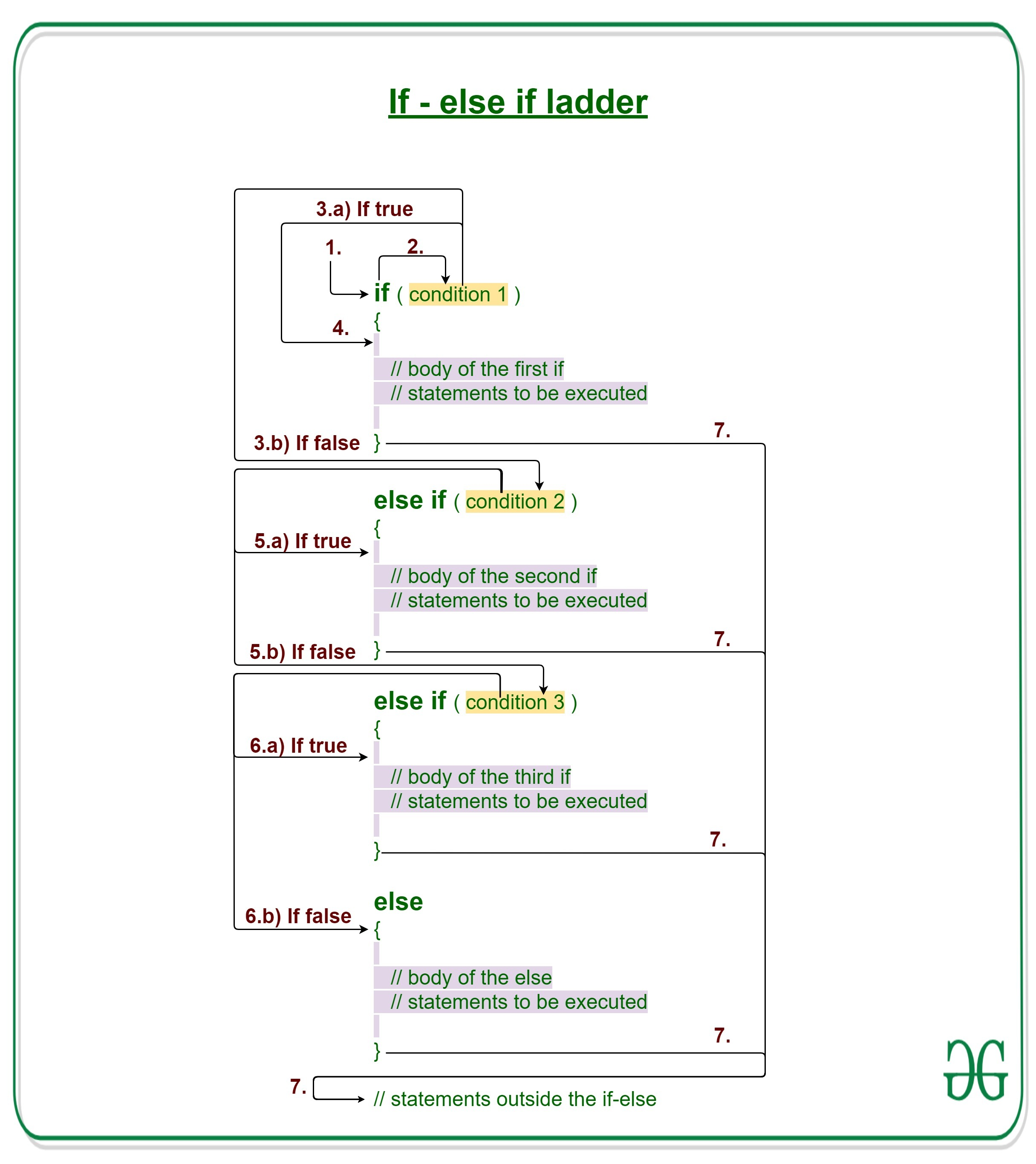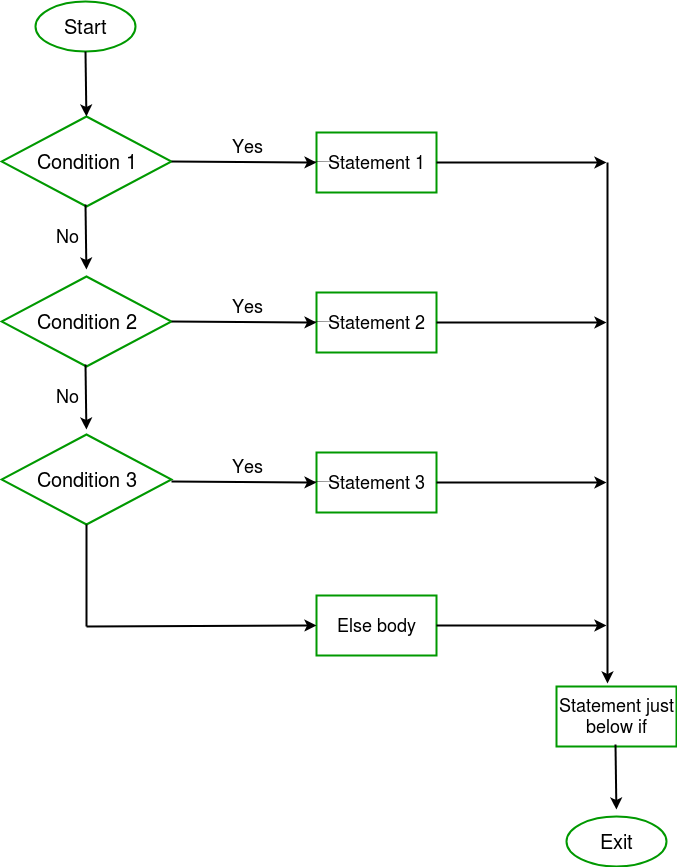# Java if-else-if ladder with Examples

Decision Making in Java helps to write decision-driven statements and execute a particular set of code based on certain conditions.
Java if-else-if ladder is used to decide among multiple options. The if statements are executed from the top down. As soon as one of the conditions controlling the if is true, the statement associated with that if is executed, and the rest of the ladder is bypassed. If none of the conditions is true, then the final else statement will be executed.Syntax:

```if (condition)
statement 1;
else if (condition)
statement 2;
.
.
else
statement;```

1. Control falls into the if block.
2. The flow jumps to Condition 1.
3. Condition is tested.
1. If Condition yields true, goto Step 4.
2. If Condition yields false, goto Step 5.
4. The present block is executed. Goto Step 7.
5. The flow jumps to Condition 2.
1. If Condition yields true, goto step 4.
2. If Condition yields false, goto Step 6.
6. The flow jumps to Condition 3.
1. If Condition yields true, goto step 4.
2. If Condition yields false, execute else block.
Goto Step 7.Example 1:

## Java

 `// Java program to illustrate if-else-if ladder`   `import` `java.io.*;`   `class` `GFG {` `    ``public` `static` `void` `main(String[] args)` `    ``{` `        ``// initializing expression` `        ``int` `i = ``20``;`   `        ``// condition 1` `        ``if` `(i == ``10``)` `            ``System.out.println(``"i is 10\n"``);`   `        ``// condition 2` `        ``else` `if` `(i == ``15``)` `            ``System.out.println(``"i is 15\n"``);`   `        ``// condition 3` `        ``else` `if` `(i == ``20``)` `            ``System.out.println(``"i is 20\n"``);`   `        ``else` `            ``System.out.println(``"i is not present\n"``);`   `        ``System.out.println(``"Outside if-else-if"``);` `    ``}` `}`

Output:

```i is 20

Outside if-else-if```

Time Complexity: O(1)

Auxiliary Space: O(1)

Dry running

Example 1:

```1. Program starts.
2. i is initialized to 20.
3. condition 1 is checked. 20 == 10, yields false.
4. condition 2 is checked. 20 == 15, yields false.
5. condition 3 is checked. 20 == 20, yields true.
5.a) "i is 20" gets printed.
6. "Outside if-else-if" gets printed.
7. Program ends.```

Example 2:

## Java

 `// Java program to illustrate if-else-if ladder`   `import` `java.io.*;`   `class` `GFG {` `    ``public` `static` `void` `main(String[] args)` `    ``{`   `        ``// initializing expression` `        ``int` `i = ``20``;`   `        ``// condition 1` `        ``if` `(i < ``10``)` `            ``System.out.println(``"i is less than 10\n"``);`   `        ``// condition 2` `        ``else` `if` `(i < ``15``)` `            ``System.out.println(``"i is less than 15\n"``);`   `        ``// condition 3` `        ``else` `if` `(i < ``20``)` `            ``System.out.println(``"i is less than 20\n"``);`   `        ``else` `            ``System.out.println(``"i is greater than "` `                               ``+ ``"or equal to 20\n"``);`   `        ``System.out.println(``"Outside if-else-if"``);` `    ``}` `}`

Output:

```i is greater than or equal to 20

Outside if-else-if```

Time Complexity: O(1)

Auxiliary Space: O(1)

Related Articles:

Whether you're preparing for your first job interview or aiming to upskill in this ever-evolving tech landscape, GeeksforGeeks Courses are your key to success. We provide top-quality content at affordable prices, all geared towards accelerating your growth in a time-bound manner. Join the millions we've already empowered, and we're here to do the same for you. Don't miss out - check it out now!

Previous
Next